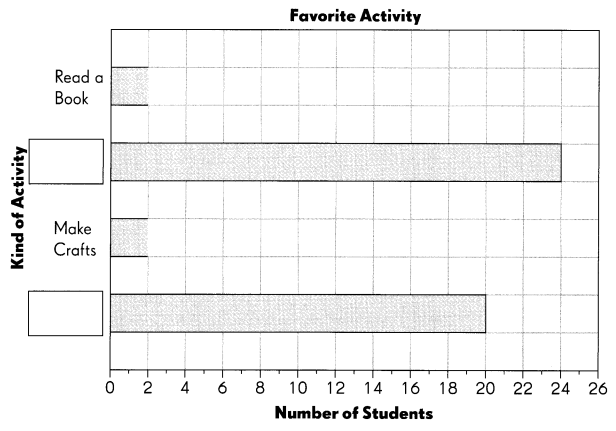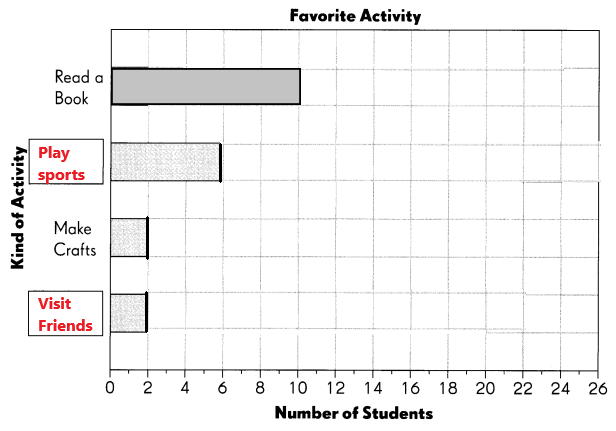# Math in Focus Grade 3 Chapter 13 Practice 1 Answer Key Making Bar Graphs with Scales

Practice the problems of Math in Focus Grade 3 Workbook Answer Key Chapter 13 Practice 1 Making Bar Graphs with Scales to score better marks in the exam.

## Math in Focus Grade 3 Chapter 13 Practice 1 Answer Key Making Bar Graphs with Scales

The picture graph shows the number of each kind of kite some students made after school.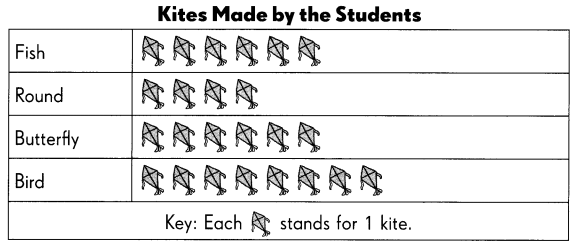Talya used the data from the picture graph to make a bar graph. She used a scale of 2.

Help Talya complete the bar graph.

Question 1.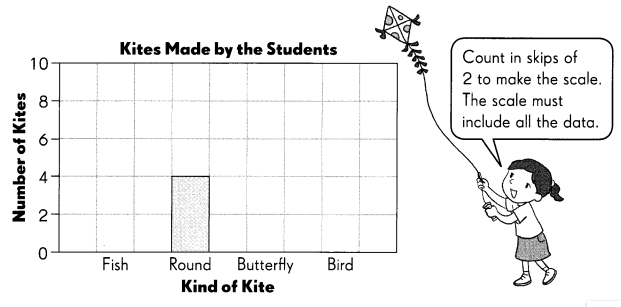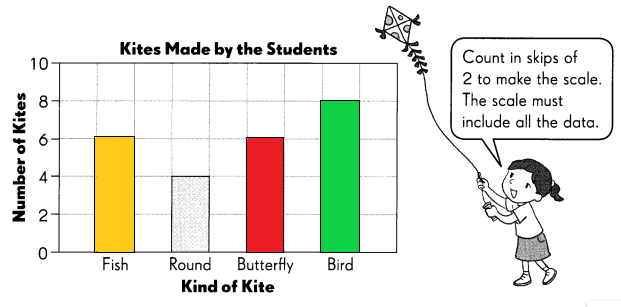Alice went to a bird park and saw 5 kinds of birds. She recorded the number of each kind of bird she saw in a tally chart.

Complete the tally chart.

Question 2.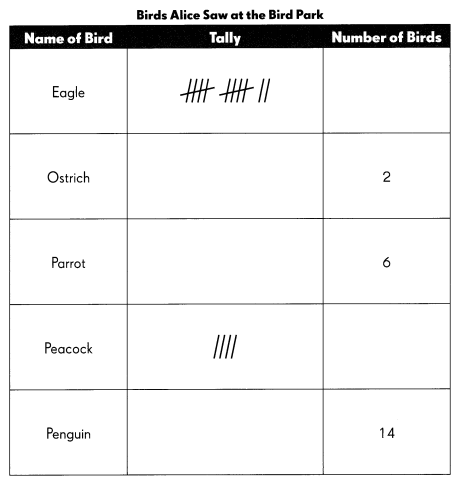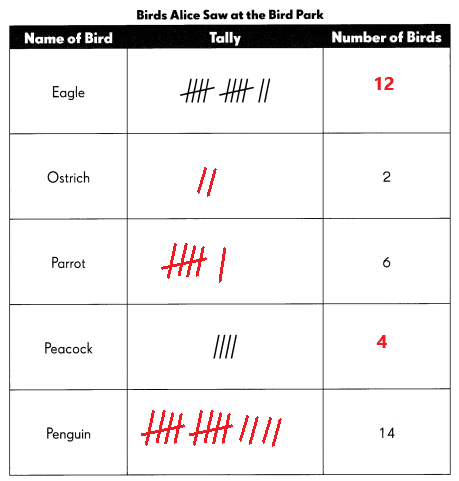Complete the bar graph to show the birds Alice saw.

Question 3.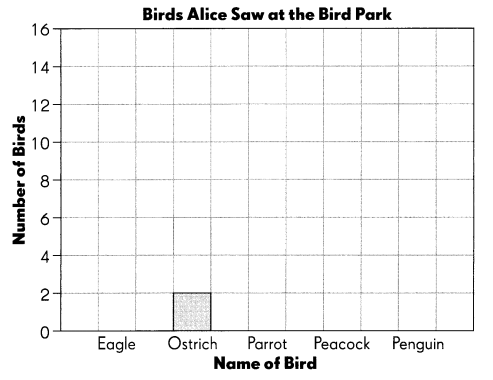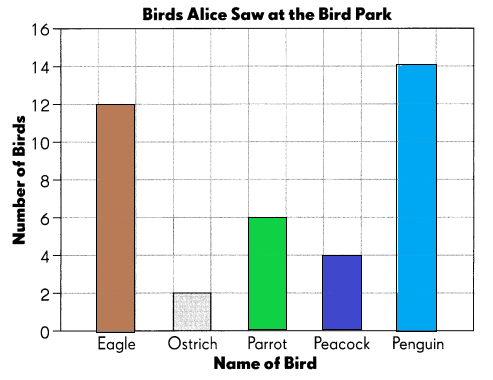Answer each question. Use the data in the bar graph.

Question 4.
The scale shows skip counts of __________.
The scale shows skip counts of 2.
Explanation:
Count in skips of 2 to make the scale. The scale must include all the data.

Question 5.
What is the greatest number on the vertical axis? Explain why.
The greatest number on the vertical axis is 16. Alice saw 14 penguin birds at the bird park. So, I taken the next value 16 on the vertical axis.

Joy and her friends are making animal masks. Count the number of each type of mask they have made.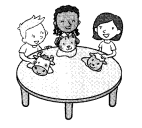Complete the tally chart and bar graph on page 65.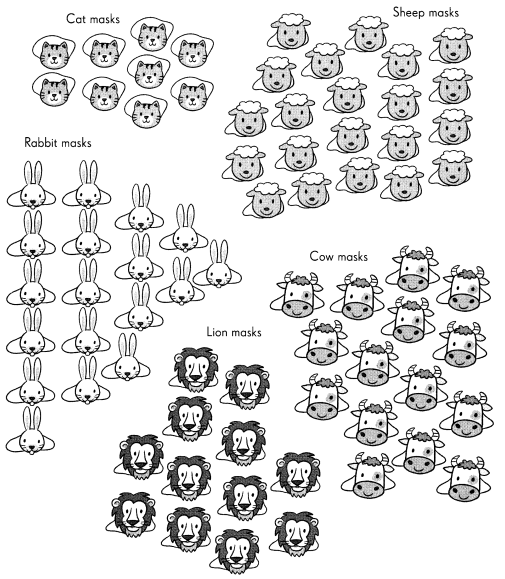Complete.

Question 6.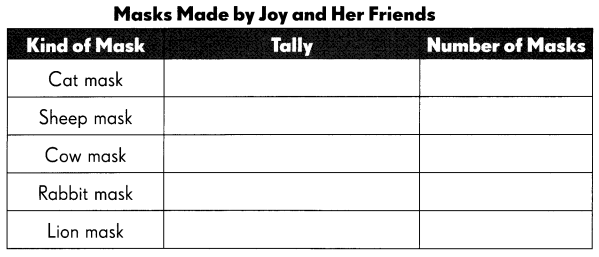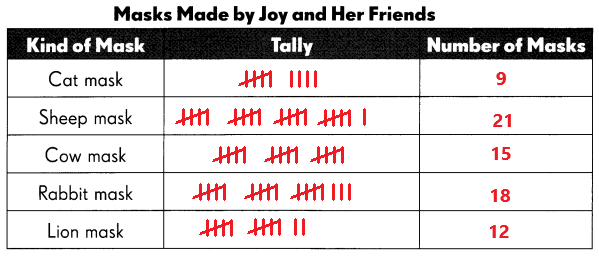Complete the bar graph. Use the data in the tally chart.

Question 7.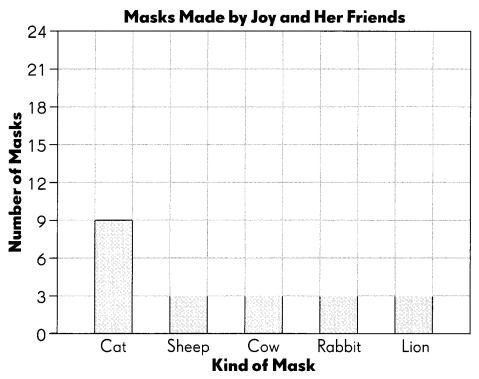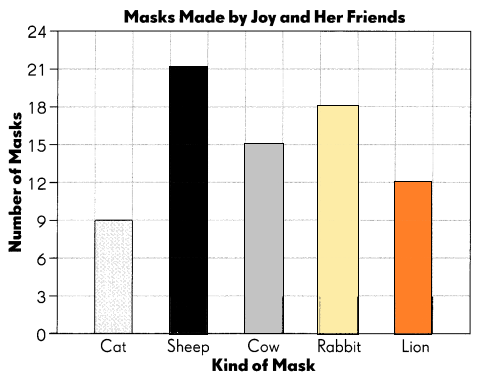Answer each question. Use the data in the bar graph.

Question 8.
The scale shows skip counts of __________.
The scale shows skip counts of 3.

Question 9.
What is the greatest number on the scale?
The greatest number on the scale is 24.

The picture graph shows the number of points five players scored in a basketball game.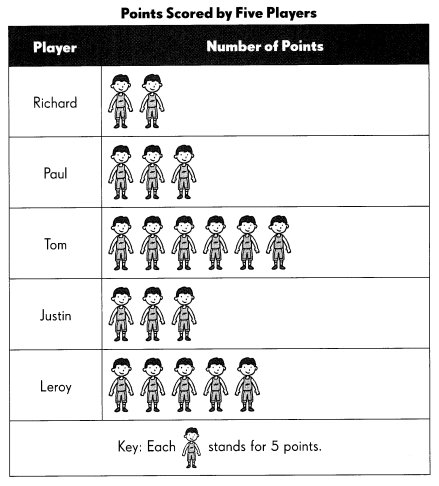Use the data in the picture graph to complete the bar graph.

Question 10.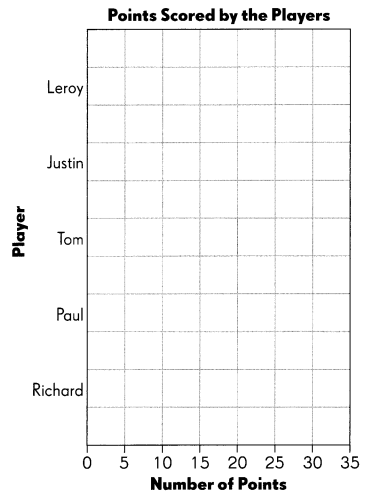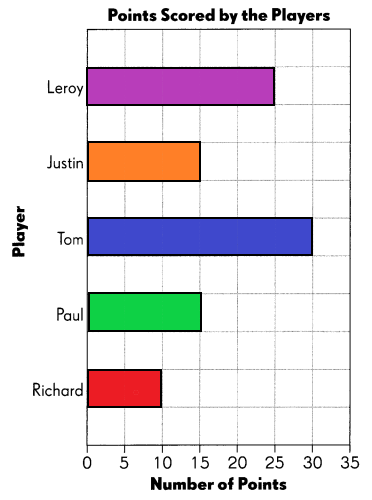Answer each question. Use the data in the bar graph.

Question 11.
The scale shows skip counts of ___________.
The scale shows skip counts of 5.

Question 12.
What is the greatest number on the scale?
The greatest number on the scale is 35.

A survey was carried out to find the favorite activities of third graders.
It was found that …
10 like to read a book.
2 like to make crafts.
2 times as many children like to play sports as make crafts.
4 fewer children like to visit friends than play sports.

Complete the bar graph to show the favorite activities of third graders. Then fill in the missing activity names in the answer boxes.

Question 13.